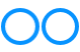# John Errington's Experiments with an Arduino

## Voltage measurement with the Arduino board (cont).

When measuring a voltage external to your system - for example logging computer power supply voltages - an accurate reference voltage is essential.  However when making measurements within your own system this may not be necessary.
Lets look at some different approaches.

measuring a voltage within the range of the ADC

measuring a proportion of the supply voltage

measuring an external voltage that is more than the adc reference voltage

measuring an external voltage using a reference voltage

measuring other external voltages

### 1: the simplest case: measuring a voltage within the range of the ADC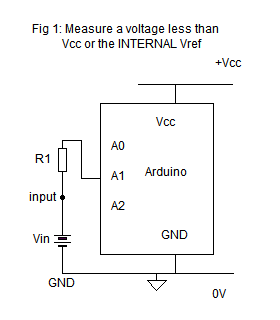Suppose you are using a UNO and have chosen "DEFAULT" (i.e. Vcc "5V") as your reference.

Provided that Vin is NEVER less than 0V or more then Vcc you CAN just connect your input voltage to an ADC pin - shown here A1.

However if the input happens to exceed those limits the ADC or even the board could be damaged. Its safer to add a "protection resistor" as shown here (R1).

The value should be chosen to limit the current to less than 1mA so for most purposes a 10k resistor will be fine.

(OK unless its connected to the mains or struck by lightning!)

### 2: measuring a proportion of the supply voltage

Here we have no problems. Suppose you are using a simple potentiometer type sensor, like you find in a joystick or gamepad. Moving the joystick moves a sliding contact that taps off a fraction Vp of the applied voltage (see diagram).

Because we are measuring a fraction of the supply we dont need to worry about exceeding those voltage limits - it cant happen.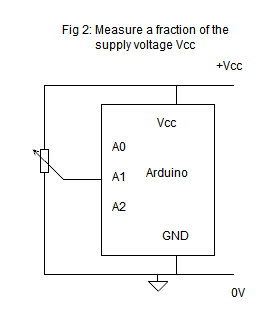Suppose the potentiometer is set to 1/4 of its travel.

When the USB supply voltage is exactly 5V the voltage from the pot. will be
Vpot = 1/4 * 5V = 1.25V
Using the USB supply as reference this gives a reading of
n = 1.25V / 5V * 1024 = 256

If the USB supply falls to 4.40V the voltage from the pot changes to Vpot = 1/4 * 4.40 = 1.10V
Using the supply as reference this gives a reading of
n = 1.10V / 4.40V * 1024 = 256.

You can see the value of the supply voltage has no effect on the sensor reading.

### 3:  measuring an external voltage that is more than the adc reference voltage

We can use the same idea of a potentiometer to scale down voltages above the reference voltage so that the Arduino can measure them.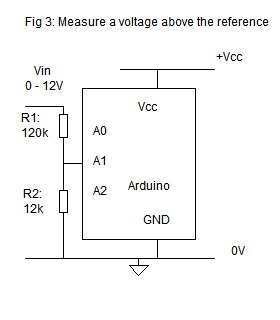Arduino models using the ATmega168 or ATmega328 have an internal reference of 1.1V.

This circuit shows how you can use an external resistor divider to scale a 12V input to "about" 1.1V

which you can then measure against the internal Vref.

The internal reference is not very precise, so you may prefer to use an external reference as described below.

### 3b:  measuring an external voltage using a reference voltage

We may wish to use an external voltage reference for good accuracy. The diagram shows an Arduino used to measure voltages in the range 0 - 10V, using a potential divider network R1: R2, and an external voltage reference of 3.000V.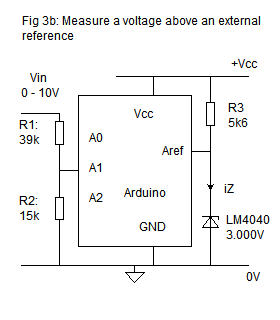According to the data sheet
"The ADC is optimized for analog signals with an output impedance of 10k or less. .. . The user is recommended to only use low impedance sources with slowly varying signals, since this minimizes the required charge transfer to the S/H capacitor."  (2: 26.6.1)

This requirement is ONLY a concern if your measured voltage is changing rapidly (say more than 50Hz).

For this application we choose a divider network that will provide a source of around this impedance. (39k //15k = 11k)

Here we are using a 3.000V reference, which gives a current of (5V - 3V) / 5k6 = 360uA.

3V/32k = 94uA flows through the internal resistance of the Aref input, leaving 266uA for the reference.

To calculate the input voltage Vin from the ADC reading you will need to know the exact values of R1 and R2, or calibrate your circuit as described on this page.

### 4: measuring other external voltages

For positive voltages we use the same circuit as above, but simply change the divider chain. Lets design a circuit to measure in the range 0 - 30V. The divider needs to present a voltage of 0 - 3V at A0, so for a 30V input we will have 3V across R2 and 30 - 3 = 27V across R1. We need a ratio R1:R2 of 27:3, such that R1 // R2 = 10k.

Choosing R2 = 11k and R1 = 100k gives the range we need, with a source resistance of 9.9k

Acknowledgements: All diagrams drawn with MeeSoft Diagram Designer